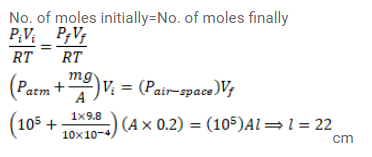Deepak Scored 45->99%ile with Bounce Back Crack Course. You can do it too!

# Use R=8.3 J/mol-K wherever required. An ideal gas is kept in a long cylindrical vessel

Question:

Use $\mathrm{R}=8.3 \mathrm{~J} / \mathrm{mol}-\mathrm{K}$ wherever required.

An ideal gas is kept in a long cylindrical vessel fitted with a frictionless piston of cross-sectional area 10 $\mathrm{cm}^{2}$ and weight $1 \mathrm{~kg}$. The length of the gas column in the vessel is $20 \mathrm{~cm}$. The atmospheric pressure is $100 \mathrm{kPa}$. The vessel is now taken into a spaceship revolving round the earth as a satellite. The air pressure in the spaceship is maintained at $100 \mathrm{kPa}$. Find the length of the gas column in the cylinder.

Solution: